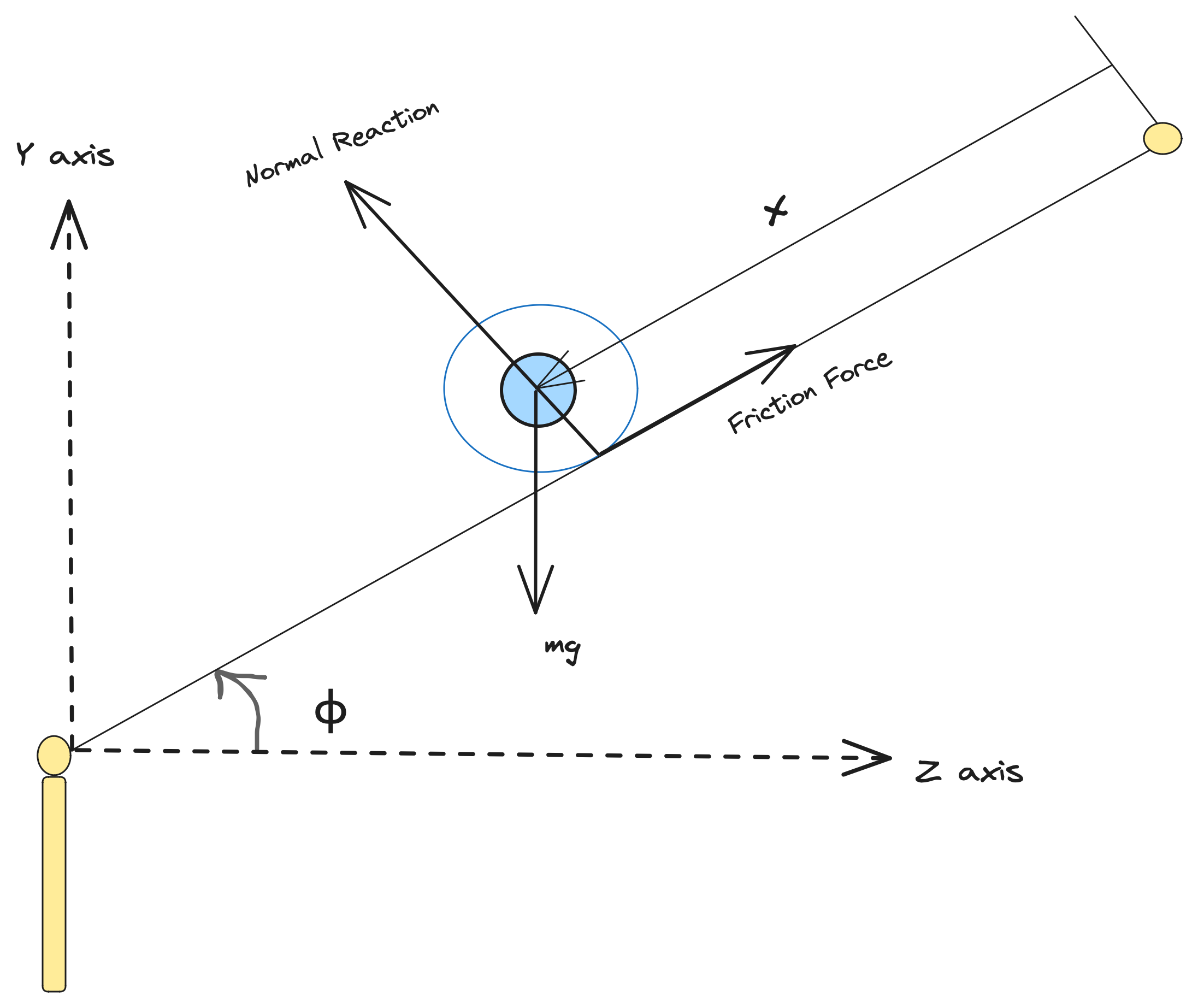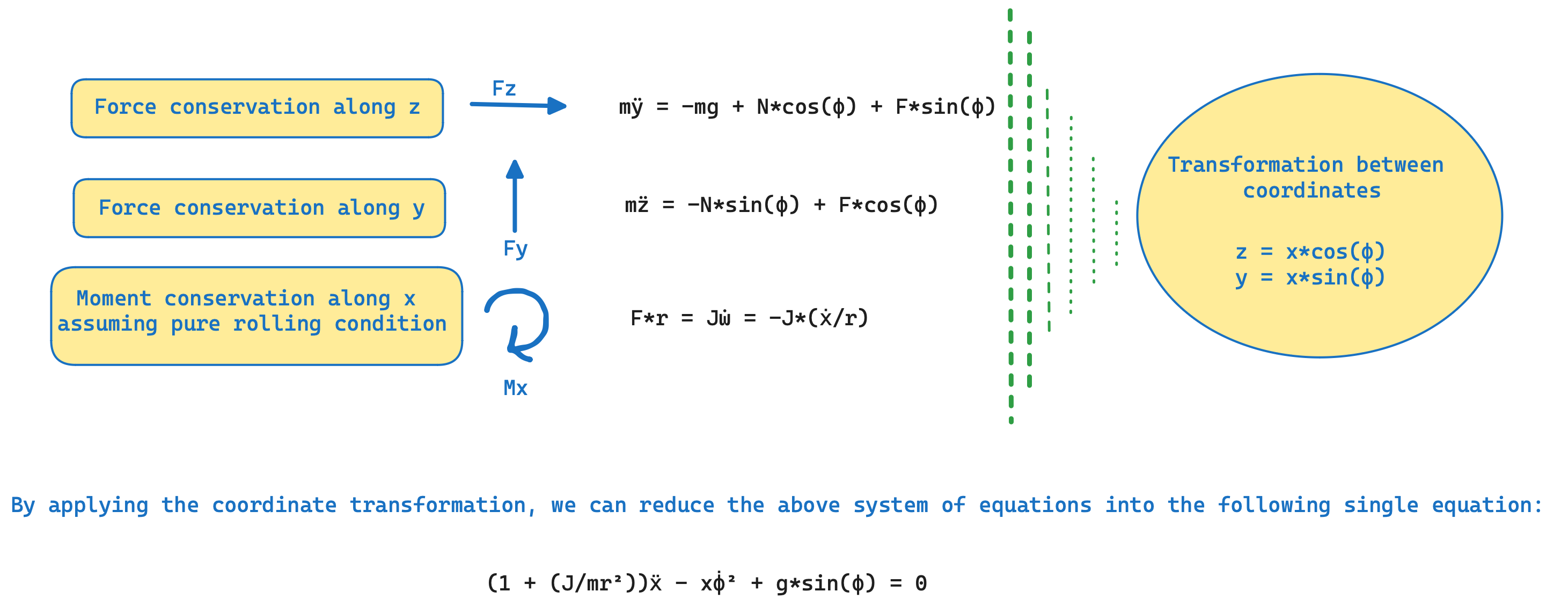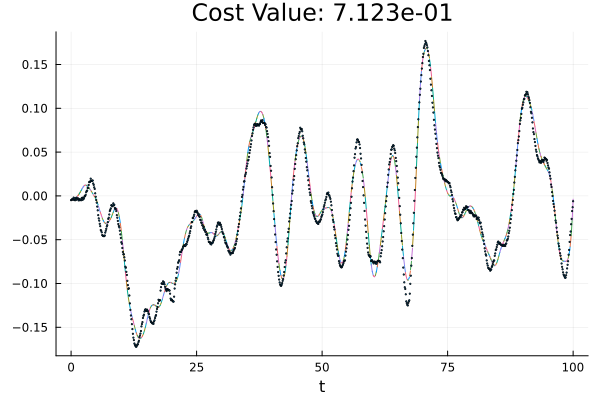# Parametric Uncertainty Quantification in an Unstable Dynamical System

Parametric uncertainty is an important type of uncertainty which arises due to imprecision or lack of proper knowledge about the true values of the model parameters. In general often we are interested in evaluating the uncertainty distributions of the parameters, based on the available input data and investigating how well are we able to recover the parameters based on the goodness of the data-fit. In the Getting Started with the JuliaSimModelOptimizer we used the calibrate method to solve an inverse problem. This was achieved by finding a single combination of parameters that fit the data well. Often, multiple parameter combinations fit the data (almost) equally well. One single estimate from a calibration can give the wrong impression about the uncertainty present in the model parameters. parametric_uq is another method to solve inverse problems, that tries to remedy this issue. Contrary to calibrate, this method returns multiple combinations of parameters that are plausible given the observed data. One algorithm that can be used with this function is StochGlobalOpt, which starts multiple optimizations from a latin hypercube sampling of the search space.

In this tutorial we show how can one use parametric uncertainty quantification for an unstable dynamical system, namely the "ball and beam" system. As shown in the schematic below, in this case, our process model involves, a horizontal beam and a motor. The control objective is to balance a rolling ball on the beam, when the position of the ball changes, by controlling the beam angle using a motor. Here, the system has three parameters namely ${I, g, f_v}$, where I stands for the non-dimensional parameter, ${J/(m*r^2)}$, which is analytically 0.4 in this case for the spherical-ball, g stands for the acceleration due to gravity, and ${f_v}$ stands for the Coulomb's friction coefficient parameter, with all units taken in S.I. Assuming there is negligible dynamics inside the motor components, hence the angular velocity of beam, is directly proportional to the input. The dynamical equations of the ball, based on the classical mechanics for a Coulomb's friction model, can be derived from the conservation equations described below.The dataset for this system, has been collected from the ball-and-beam experiment setup in the STADIUS's Identification Database.

The measured signals out of this process, are two of the state variables of the problem, the beam angle relative to the horizontal plane (φ) and the position of the ball (x). First, we start the problem, by defining the governing system of ODEs, that consists of the following equations:-

\begin{aligned} \frac{d\phi}{dt} &= f^n(t) \\ (1+ I) \frac{d^2x}{dt^2} - x (\frac{d\phi}{dt})^2 + f_v \frac{dx}{dt} + g sin(\phi) &= 0 \\ \end{aligned}

## Julia Environment

using JuliaSimModelOptimizer
using ModelingToolkit
using OrdinaryDiffEq
using DataFrames
using StatsPlots
import DataInterpolations: CubicSpline
import ForwardDiff
using OptimizationOptimJL
using DataSets
using CSV
using ModelingToolkitStandardLibrary.Blocks: RealInput, TimeVaryingFunction

## System Setup

training_dataset = dataset("juliasimtutorials/ball_beam_data")

data = open(IO, training_dataset) do io
end

df=DataFrame("timestamp" => range(0,100,1000),"ball_position" => data[:,2])

t_vec = df[!,"timestamp"]
ϕ_vec = data[:,1]

input_func = CubicSpline(ϕ_vec,t_vec)

dinput = t_vec -> ForwardDiff.derivative(input_func,t_vec)
dinput_vec = dinput.(t_vec)
dinput_func = CubicSpline(dinput_vec,t_vec)

pos_func = CubicSpline(data[:,2],t_vec)
dpos_func = t_vec -> ForwardDiff.derivative(pos_func,t_vec)
dpos_vec = dpos_func.(t_vec)

function ballandbeamsystem()

iv = only(@variables t)
states = @variables x(t) = -4.8851980e-03 ϕ(t) = -1.9634990e-03
@variables ball_position(t)
ps = @parameters I = 0.2 g = 9.8 Fv = 1.0
D = Differential(t)
@named input = RealInput()

eqs = [
D(ϕ) ~ input.u,
0 ~ (1 + I) * D(D(x)) - x * (D(ϕ))^2 + g * sin(ϕ) + Fv * D(x),
]

obs_eq = [ball_position ~ x]

return ODESystem(eqs, iv, states, ps, systems=[input];
observed = obs_eq,
name = :ballandbeamsystem)
end

function SystemModel(; name=:model)
f = dinput_func

bbsys = ballandbeamsystem()
t = ModelingToolkit.get_iv(bbsys)
src = TimeVaryingFunction(f; name=:src)
eqs = [ModelingToolkit.connect(bbsys.input, src.output)]

ODESystem(eqs, t; systems=[bbsys, src], name)
end

model = complete(SystemModel())   #ballandbeamsystem()
missing_sys = structural_simplify(model)
complete_sys = missing_sys
@unpack iv, x, ϕ, ball_position, I, g, Fv = model.ballandbeamsystem
\begin{align} \frac{\mathrm{d} \phi\left( t \right)}{\mathrm{d}t} =& input_{+}u\left( t \right) \\ 0 =& Fv \frac{\mathrm{d} x\left( t \right)}{\mathrm{d}t} + g \sin\left( \phi\left( t \right) \right) + \left( 1 + I \right) \frac{\mathrm{d}}{\mathrm{d}t} \frac{\mathrm{d} x\left( t \right)}{\mathrm{d}t} - \left( \frac{\mathrm{d} \phi\left( t \right)}{\mathrm{d}t} \right)^{2} x\left( t \right) \end{align}

The data that we have for the system corresponds to the angle of the beam relative to the horizontal plane (φ) and the position of the ball on the beam (x).

## Defining the Experiment from the imported data

In order to create the custom experiment, we will use the default initial values of the states and parameters of our model. These are our initial guesses which will be used to optimise the inverse problem in order to fit the given data. Also, in this system, we need to pass the un-initialised dummy derivatives terms to the existing initialised variable map.

push!(complete_sys.defaults,ModelingToolkit.missing_variable_defaults(missing_sys, [dpos_vec]))
rename!(df, :ball_position => Symbol("ballandbeamsystem.ball_position"))
experiment = Experiment(df, complete_sys)
Experiment with default parameters and initial conditions.
timespan: (0.0, 100.0)


## Generating possible solutions for the inverse problem

Once we have created the experiment, the next step is to create the problem. This inverse problem, requires us to provide the search space as a vector of pairs corresponfing to the parameters that we want to recover and the assumption that we have for their respective bounds.

prob = InverseProblem(experiment,
complete_sys,
[model.ballandbeamsystem.I => (0, 1), model.ballandbeamsystem.Fv => (0, 2)])

alg = StochGlobalOpt(
method = MultipleShooting(
optimizer = BFGS(),
trajectories = 500,
maxiters = 10^3,
maxtime = 2000),

ps = parametric_uq(prob, alg, sample_size = 10)
Parametric uncertainty ensemble of length 10 computed in 37 minutes and 27 seconds.
┌─────────────────────┬──────────────────────┐
│ ballandbeamsystem₊I │ ballandbeamsystem₊Fv │
├─────────────────────┼──────────────────────┤
│            0.616876 │             0.860185 │
│            0.473667 │              1.58273 │
│            0.688556 │             0.370203 │
│            0.537997 │              1.35491 │
│            0.530797 │             0.762647 │
│            0.452527 │              1.18959 │
│            0.863463 │              1.63974 │
│            0.360327 │             0.537312 │
│            0.726737 │              1.09822 │
│            0.578666 │              1.86207 │
└─────────────────────┴──────────────────────┘


## Analysis of the results

confidence_plot_shooting_segments(experiment, ps, confidence = 0, ms = 0.8)The confidence_plot_shooting_segments represents a comparision plot between the actual data and the simulated experiment that is solved in each of the time segments, based on the level of confidence amongst the generated set of parameters. The keyword argument confidence = 0, signifies the data fit for the worst fitted parameter set.